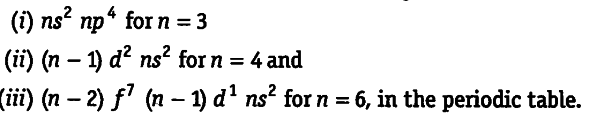# Assign the position of the element having outer electronic configuration

Assign the position of the element having outer electronic configuration.(i) \${{ns}^{2}}\$ \${{np}^{4}}\$ for n = 3
n = 3 means element belongs to third period. Since, last electron enters in the p-orbital, it belongs to p-block. For p-block elements, the group number = 10 + valence shell electrons = 10 + (2 + 4) = 16. Hence, the element belongs to 16th group.
(it) (n — 1) \${{d}^{2}}\$ \${{ns}^{2}}\$ for n = 4
n = 4 means the element belongs to fourth period. Since, last electron enters in d-orbital, the given element belongs to d-block. For d-block elements, group number = number of d-electrons + number of ns electrons = 2 + 2 = 4
Hence, the element belongs to 4th group.
(in) (n — 2) \${{f}^{7}}\$ (n — l)\${{d}^{1}}\$ \${{ns}^{2}}\$ for n = 6
n = 6 means, the element belongs to sixth period.
Since, last electron enters in /-orbital, the given element belongs to f-block and all f-block elements are the members of third group. Hence, the element belongs to third group.